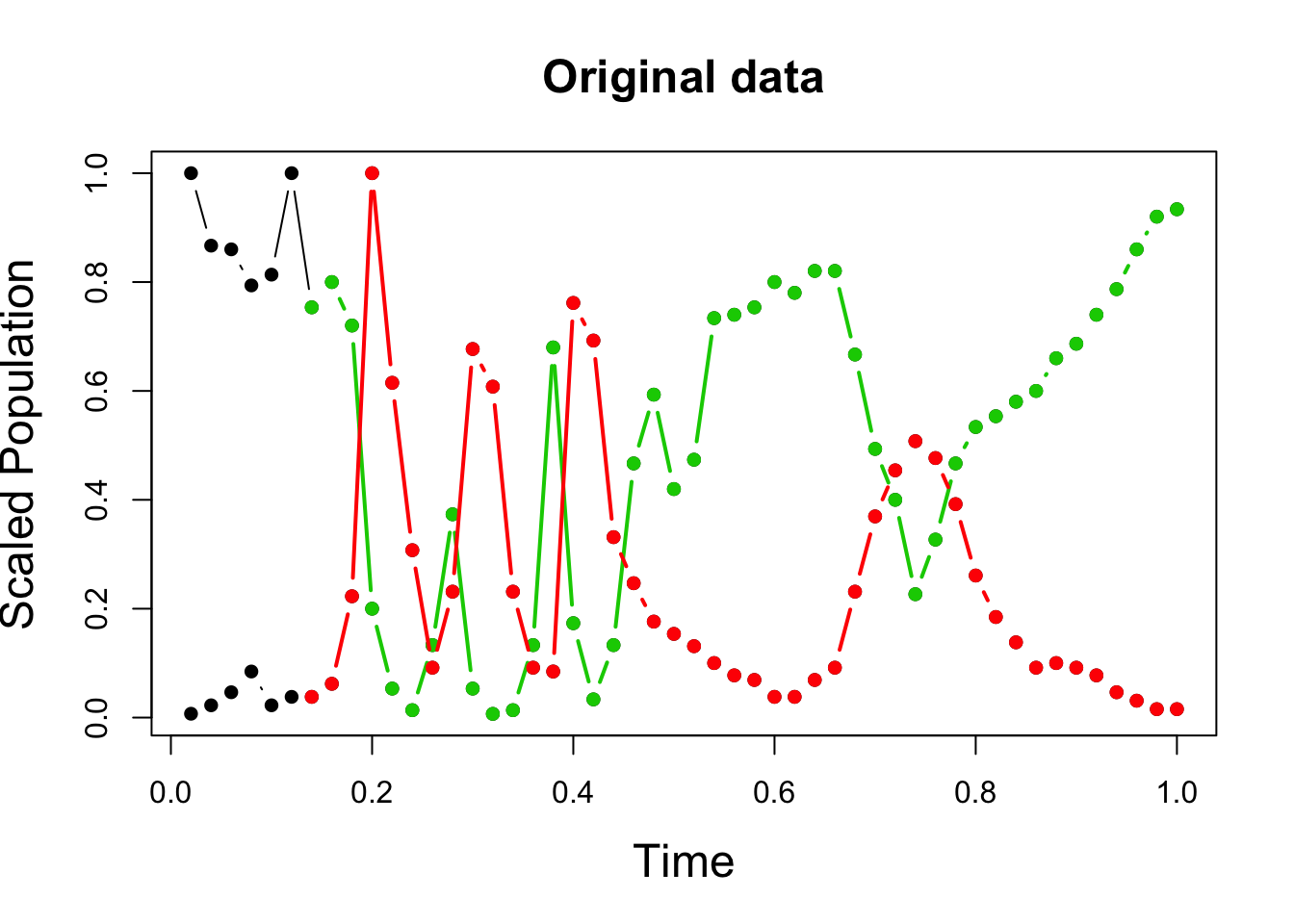# Introduction

Here we will reproduce the time series anaylses presented in the paper A newly discovered role of evolution in previously published consumer-resource dynamics, by Tepo Hiltunen and others. The manuscript can be found on (the paper on the Ecology Letters website).

The Authors of this paper developed a statistical method to probe for a transition from classical to eco-evolutionary cycles in consumer-resource cycles.

Classic ecological consumer-resource models often produce cyclical dynamics, where the consumerabundance lags behind the resource by ¼ of the period.There are however few published examples of experimental systems showing this pattern.

In this study, the authors reveal that many observed and published consumer-resource dynamics show instead patterns indicating eco-evolutionary dynamics.Theoretically, if prey evolve along a trade-off between defence and competitive ability, two-species consumer-resource cycles become longer and antiphase(half-period lag, so consumer maxima coincide with minima of the resource species). Such antiphase patterns of eco-evolutionary cycles take time to emerge, as prey must evolve to a defended state.The authors applied thir analysis to 21 two-species consumer-resource time series, published between 1934 and 1997.They aimed to statistically identify a transition from ecological dynamics at initial point of experiment (¼ lag as ), to eco-evolutionary cycles.

# The data

The time series data sets analysed are provided in the the Supplement to the paper. The data contains a set of 12 predator-prey time series. Each predator-prey time series is in a seperate Excel spreadsheet. Each data file details the timing of predator and prey obserations, the abundance of each observed, the start and end time of the predator prey oscilations and the dilution of medium in the system. A script detailing the data transfromation and smoothing methods are also provided in the supplementary materials.

Although all data sets are analysed in this study, the Amoeba-bacteia predator-prey data set of Tsuchiya et al. (1972) was the focus of the figures in the manuscript. Therefore we also use this data set to perform the reproduction

We thank and aknowledge Steve ellner for help in the reproduction of this work.

# First get the raw data into R and clean it.

``````rm(list=ls())
require("RColorBrewer")``````
``## Loading required package: RColorBrewer``
``library(RCurl)``
``## Loading required package: bitops``
``````# load the data from github
x <-  getURL("https://raw.githubusercontent.com/opetchey/RREEBES/master/Hiltunen_etal_2014_EcologyLetters/data/Tsuchiya%201972%20set%20C.csv",
cainfo = system.file("CurlSSL", "cacert.pem", package = "RCurl"))

Note that the time of the predator and prey observations may differ. Also the 5th to 10th column of data contains just a single value and all the other elenments are NA.This reflects the fact that they are not part of the time series data, but are instead constants to use in the analysis.

``````n.obs <- dim(pp.dat) # total number of observations
ww=pp.dat\$window;    # window length as percent of total.
start_cut= pp.dat\$start #how much to remove from the start and end
end_cut=pp.dat\$end ``````

Assign prey and predator observations and remove any NaNs

``````preyX=pp.dat\$prey_time; preyY=pp.dat\$prey_pop;
Q=cbind(preyX,preyY); Q=na.omit(Q);
preyX=Q[,1]; preyY=Q[,2];

predX=pp.dat\$pred_time; predY=pp.dat\$pred_pop;
Q=cbind(predX,predY); Q=na.omit(Q);
predX=Q[,1]; predY=Q[,2];``````

Scale prey and predator number relative to maximum

``````preyX=preyX/max(preyX); preyY=preyY/max(preyY)
predX=predX/max(predX); predY=predY/max(predY)``````

Join data back together and cut out initial transient

``````prey1=cbind(preyX,preyY)
prey1=data.frame(prey1)
prey_all=data.frame(prey1)
prey1=prey1[prey1\$preyX>start_cut,];
prey1=prey1[prey1\$preyX<end_cut,];

pred1=cbind(predX,predY)
pred1=data.frame(pred1)
pred_all=data.frame(pred1)
pred1=pred1[pred1\$predX>start_cut,];
pred1=pred1[pred1\$predX<end_cut,];``````

# Plot the time series (rescaled)

``````xmin=max(min(pred1\$predX,na.rm=T),min(prey1\$preyX,na.rm=T)); xmax=min(max(pred1\$predX,na.rm=T),max(prey1\$preyX,na.rm=T));
yrange=range(c(pred_all\$predY,prey_all\$preyY));

plot(prey_all,type='b',col = "black",xlab="Time", ylab="Scaled Population",xlim=c(min(prey_all[,1]), 1),pch=16,ylim=yrange,cex.lab=1.5)
lines(pred_all, type='b',col = "black",ylim=yrange,xlim=c(min(prey_all[,1]), 1),pch=16)
lines(prey1, type='b',col = "green3",lwd=2,ylim=yrange,xlim=c(min(prey_all[,1]), 1),pch=16)
lines(pred1, type='b',col = "red",,lwd=2,ylim=yrange,xlim=c(min(prey_all[,1]), 1),pch=16)
title(paste("Original data"),cex.main=1.5)``````# Transform and smooth the data

Transform scaled abundance data (log)

``````##log 10 both pred and prey abundances
prey1\$preyY=log10(prey1\$preyY); pred1\$predY=log10(pred1\$predY); ``````

Peform smoothing: constrain df of spline to be <= 2/3 of time series length

``````smooth_prey=smooth.spline(prey1\$preyX,prey1\$preyY);
maxdf=0.67*length(prey1\$preyX);
if(smooth_prey\$df>maxdf) smooth_prey=smooth.spline(prey1\$preyX,prey1\$preyY,df=maxdf);

smooth_pred=smooth.spline(pred1\$predX,pred1\$predY);
maxdf=0.67*length(pred1\$predX);
if(smooth_pred\$df>maxdf) smooth_pred=smooth.spline(pred1\$predX,pred1\$predY,df=maxdf); ``````

Predict the smoothed values of the abundances over the time of observation

``````#seq of scaled times from min to max by 100
px.prey=seq(min(prey1[, 1]),max(prey1[, 1]),length=100);
px.pred=seq(min(pred1[, 1]),max(pred1[, 1]),length=100);

smooth_pred2=predict(smooth_pred,px.pred)\$y;
smooth_prey2=predict(smooth_prey,px.prey)\$y; ``````

# Plot transformed & smoothed time series

Plot data on log scale, with smooths (figure 1a)

``````ylims=range(c(prey1\$preyY,pred1\$predY));

plot(px.prey,smooth_prey2, type='l',xlab="Scaled time", ylab="log10(Scaled Populations)",
lwd=3,col = "green3", ylim=ylims,xlim=c(min(prey_all[,1]), 1))
lines(prey1, type='p',col = "green3",lwd=3,xlim=c(min(prey_all[,1]), 1))
lines(px.pred,smooth_pred2, type='l',lwd=3, col = "red",xlim=c(min(prey_all[,1]), 1))
lines(pred1, type='p',col = "red",lwd=3,xlim=c(min(prey_all[,1]), 1))
title("Smoothed population abundances")``````